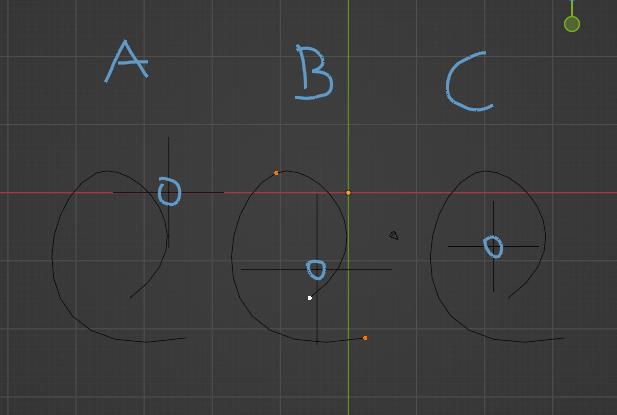# Calculating individual spline centers in a curve object

I want to compute the center of each spline from `object.data.splines`.

To do that I try something like:

``````for obj in curves:
for index in range(len(obj.data.splines)):
temp_obj = obj.evaluated_get(depsgraph).copy()
temp_obj.data = temp_obj.to_curve(depsgraph).copy()
# Remove all splines but the current one based on the `index` taken from `obj.data.splines`
for temp_spline in chain(temp_obj.data.splines[:index], temp_obj.data.splines[index + 1:]):
temp_obj.data.splines.remove(temp_spline)
temp_mesh = temp_obj.to_mesh()
# Compute mean of `temp_mesh.vertices`
temp_obj.to_mesh_clear()
temp_obj.to_curve_clear()
bpy.data.curves.remove(temp_obj.data)
``````

This doesn’t work though because `temp_obj.to_mesh()` seems to rely on some cached or something based on the splines I remove.

This results in `WARN (bke.anim): source/blender/blenkernel/intern/anim_path.c:68 BKE_anim_path_calc_data: No bev list data!` after which the spline data gets removed so `to_mesh()` fails and results in a mesh with no vertices for any `index > 0`.

In the worst case scenario I have to reconstruct a curve object with a new spline and copy all the individual points taking into account the curve type poly - nurbs, bezier which I’d rather avoid if possible.

Any other ideas on how to get the center of splines or if there’s something I’m missing that would make my implementation work?

You can calculate it in this way:

``````import bpy

def calculate_centers_of_splines():

for curve in bpy.data.curves:

for spline in curve.splines:

if spline.type != 'BEZIER':
print('only bezier splines allowed')
continue

sum_points = [0,0,0]
for p in spline.bezier_points:
for i in range(3):
sum_points[i] += p.co

total_points = len(spline.bezier_points)
if total_points == 0:
continue

result = [0,0,0]
for i in range(3):
result[i] = sum_points[i] / total_points

print('spline:', spline, "center:", result)

calculate_centers_of_splines()
``````

Chances that you have to access an enormous amount of curves is slim, from what I see if you have more than a few thousand splines with hundreds of thousands of points then you would have to seek better ways to optimize the code (better to write internal C code within Blender and eject the data as binary file).

Your solution doesn’t take into account the shape of the spline based on bezier handles (if they’re used), that’s why I wanted to convert to mesh with `temp_obj.to_mesh()` because it takes in account all parameters, including resolution, handles etc.

Yeah this way is better, to convert it so you get the final shape.

I figured out a solution that works, I don’t really like it cause it’s definitely a hack due to caching, but it does work for my need:

``````for obj in source:
obj_data = obj.data
for index in range(len(obj.data.splines)):
temp_obj = obj.evaluated_get(depsgraph).copy()
temp_obj.data = temp_obj.data.copy()
for temp_spline in chain(temp_obj.data.splines[:index], temp_obj.data.splines[index + 1:]):
temp_obj.data.splines.remove(temp_spline)
obj.data = temp_obj.data
temp_mesh = obj.to_mesh()
obj.data = obj_data
if len(temp_mesh.vertices) > 0:
vector_mean = temp_obj.matrix_world @ sum((v.co for v in temp_mesh.vertices), Vector()) / len(temp_mesh.vertices)
# Do something with vector_mean
obj.to_mesh_clear()
temp_obj.to_mesh_clear()
bpy.data.curves.remove(temp_obj.data)
``````

Awesome.on what kind of curves can this be use ?

thanks for feedback

happy bl

For POLY or BEZIER curves unless you want to get the handles, then is only BEZIER.

can’t you find the centre with the object spec ?

happy bl

No because it has to do with how the points on average are calculated. I got it wrong at first myself and then understood the problem better.A: Curve: any object by default can have it’s origin location anywhere so you can’t be sure about which is which (unless you do `set origin to cursor etc...`)

B: Curve: If you select all points and do `set cursor to selection` this is where you get the center point. But is only based on three points as the curve has.

C: Mesh: In this converted curve to mesh, now you get all of the vertices in all positions and the result is the real deal.

This algorithm can be used on any type of curve object type: `POLY`, `BEZER` & `NURBS`. I mean to be more specific, on any spline type. Cause in a curve object you can have at the very least `POLY` & `BEZIER` at the same time.

how do you define the Source variable in little script

and is it for 2.79 or BL 3.4 ?

and is your script working only for depsgraph or can work for curves in 3D viewport too ?

thanks
happy bl

The `source` variable is just an iterator of objects (list, tuple, generator etc.) as long as they’re of `bpy.types.Object` type so for example `source = [bpy.data.objects['Cube']] # assuming the Cube object exists`.

How is it defined specifically in my script? It’s based on a drop-down property that the user uses to pic objects or collections of objects and I convert those to a list of objects like I mentioned.

Only tried with 3.4.

I don’t understand the question. It works with any object that Blender has in the database. I use `depsgraph` because I want the “evaluated” object - so objects with modifiers, shape keys etc. applied. I’m pretty sure that the evaluated objects and what we see in the 3D viewport is basically the same thing, cause what we see is the state of the evaluated objects if they have modifiers etc.